Choose Language Hide Translation Bar
Highlighted

## neural network convergence

I have raw data with three independent variables: temperature, stress and creep strain and one dependent variable: creep rate (see plot below). A colleague is doing some FEM where he needs to be able to get the values between the points in the plot, so I used the neural platform in JMP to get these for him.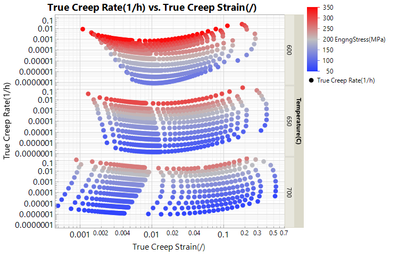This worked very well, I got very good agreement between the predictions from the neural model and and the data. I tried several models with different numbers of nodes in the hidden layer. (I don't have JMP PRO, so I can only use 1 layer.)

To decide which model (e.g. number of nodes N) to use, so I generate a plot of R2 and RMSE as a function of the number of nodes in the model. I expected that for the training set the R2 curve would rise and the RMSE curve would drop monotonously (with some random noise). For the validation set, I expected the R2 to rise and the RSME curve to drop until a certain value of N at which point the trends for the validation curve would be reversed. This would be the point at which the model would start over-fitting the training data and hence the performance on the validation data would drop.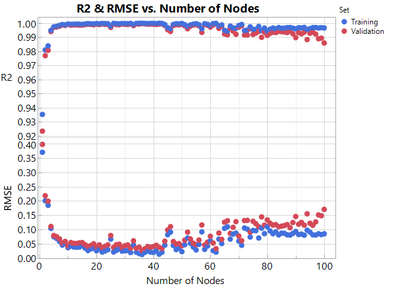Initially, the trends are pretty much as I expected, but I have some questions:

1) In a model with ca. 10 nodes or more, the RMSE for the training set is systemically lower than for the validation set. Is that already a sign for over-fitting?

2) When using more than ca. 43 nodes (for the RMSE) or ca. 63 nodes (for the R2) the performance of the model drops for the validation and the training set. Why is that? My only idea is that this might be a problem of the number of points in the data sets not being sufficient for training the neural model properly. The total data set consists of 1600 points of which I used 2/3 for the training and 1/3 for the validation set.

1 ACCEPTED SOLUTION

Accepted Solutions
Highlighted

## Re: neural network convergence

The only reference that discusses the number of hidden units is Principe, J.C., N.R. Euliano, and W.C. Lefebvre. 2000. Neural and Adaptive Systems. New York: Wiley.

My statement comes from years of experience from many SAS experts in neural networks. In one of our SAS courses about neural network essentials, we have this slide and notes: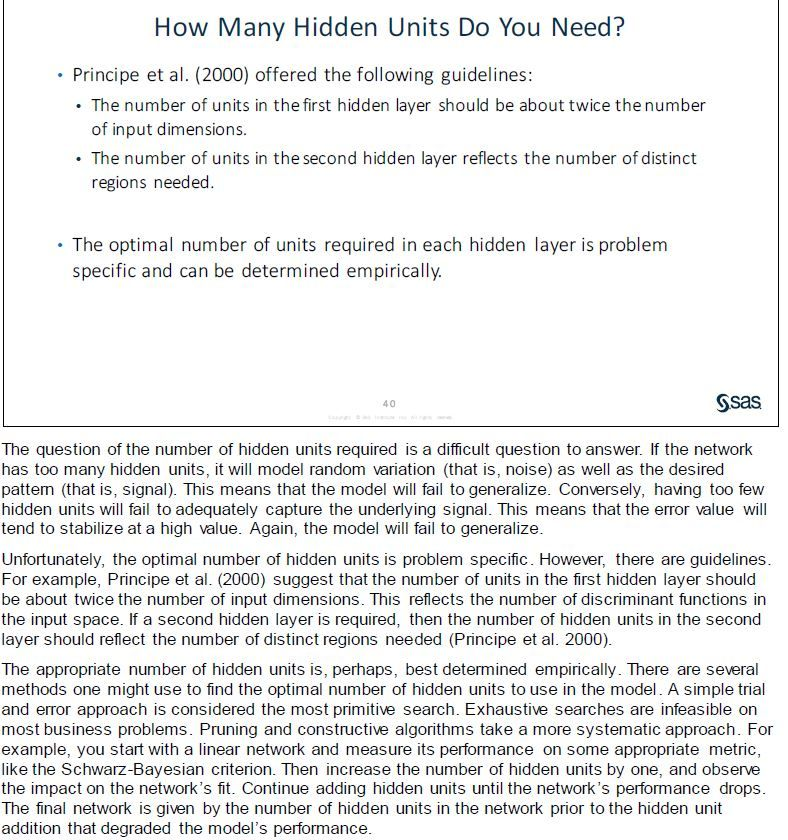Dan Obermiller
7 REPLIES 7
Highlighted

## Re: neural network convergence

Did you create and use a validation data column, or did you use the hold back feature within the platform?

Also, your response span more than a couple orders of magnitude. Did you try transforming the response, for example, with log (base 10)?

Learn it once, use it forever!
Highlighted

## Re: neural network convergence

Mark has asked very good questions. You should definitely be using a fixed validation set for these results.

Keep in mind that adding more nodes to a neural network may or may not improve predictive ability. There are many examples where the performance improves, get worse, and then improves again. Also, when you add more layers you can reach a point of node saturation which means adding more layers will not improve performance.

Dan Obermiller
Highlighted

## Re: neural network convergence

Such a neural network will only produce overfitting.

The use of deep learning convolutional neural network is a good direction.
Highlighted

## Re: neural network convergence

Thanks for your replies, I will react in a single post.

- Indeed, I used log10(strain rate) for the modelling. So what is displayed are the R2 and RSME from log10(strain rate). Sorry, I should have mentioned that.

- I use the holdback functionality to determine the validation set. However I used it with the seed 0 and the seed 123. I think if only one seed is provided, it is used for both, the determination of the starting parameters for the fitting and the selection of the validation set. (Indicating seed 0 is the same as not providing a seed.) The outcome is not fundamentally different. (There seems to be more scatter for lower number of nodes with seed 0, but the general trend looks pretty much the same.)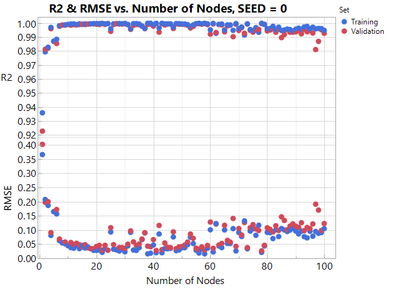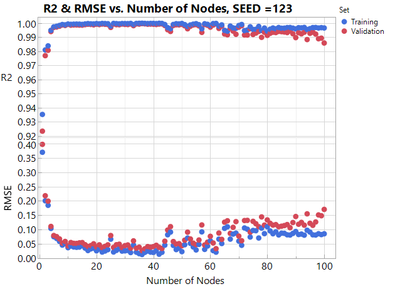@Dan_Obermiller : You mention that trends like improving, worsening, again improving performance of neural networks is often observed. Would you have some reference for this?

@lwx228: You say that "such a network will only produce overfitting". That's what I expected to see. But I expected that in the cases of overfitting, the RMSE for the validation set would rise whereas the RMSE for the training set would drop. That does not seem to be the case. (For SEED=123, the validation set has lower R2 and lower RMSE. for large number of nodes. I don't understand that...)

I add the files with the raw data and the script.

Highlighted

## Re: neural network convergence

The only reference that discusses the number of hidden units is Principe, J.C., N.R. Euliano, and W.C. Lefebvre. 2000. Neural and Adaptive Systems. New York: Wiley.

My statement comes from years of experience from many SAS experts in neural networks. In one of our SAS courses about neural network essentials, we have this slide and notes:Dan Obermiller
Highlighted

## Re: neural network convergence

One other point. I edited my original post because I confused the number of hidden layers with the number of hidden nodes. My original post now reads correctly, but since you are not using multiple layers, you can ignore the concept of node saturation.

Dan Obermiller
Highlighted

## Re: neural network convergence

Thanks. I will check that out.

Article Labels